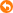本帖最後由 none_and_none 於 2019-7-29 15:13 編輯 先由砌個array入手 [[1, 2, 3, 4], [2, '', '', 5], [1, '', '', 6], [0, 9, 8, 7]] arr = 1 arr = 2 arr = 3 arr = 4 arr = 5 arr = 6 arr = 7 arr = 8 arr = 9 arr = 0 arr = 1 arr = 2 咁樣會唔會容易d諗到?
 arr = 1 arr = 2 arr = 3 arr = 4 arr = 5 arr = 6 arr = 7 arr = 8 arr = 9 arr = 0 arr = 1 arr = 2 none_and_none 發表於 2019-7-28 18:43可以見到array index係有pattern 然後就用四個loop順序去做上,右,下,左 點樣print個array出離,應該唔駛教啦掛